# Car Price Prediction Using K Nearest Neighbors¶

## Introduction¶

In this project we are going to be working on a dataset from the UCI Machine Learning Repository to make predictions on car prices. The dataset contains the following columns:

1. symboling: -3, -2, -1, 0, 1, 2, 3.
2. normalized-losses: continuous from 65 to 256.
3. make: this includes car brands such as alfa-romero, audi, bmw, chevrolet, dodge, honda etc.
4. fuel-type: diesel, gas.
5. aspiration: std, turbo.
6. num-of-doors: four, two.
7. body-style: hardtop, wagon, sedan, hatchback, convertible.
8. drive-wheels: 4wd, fwd, rwd.
9. engine-location: front, rear.
10. wheel-base: continuous from 86.6 120.9.
11. length: continuous from 141.1 to 208.1.
12. width: continuous from 60.3 to 72.3.
13. height: continuous from 47.8 to 59.8.
14. curb-weight: continuous from 1488 to 4066.
15. engine-type: dohc, dohcv, l, ohc, ohcf, ohcv, rotor.
16. num-of-cylinders: eight, five, four, six, three, twelve, two.
17. engine-size: continuous from 61 to 326.
18. fuel-system: 1bbl, 2bbl, 4bbl, idi, mfi, mpfi, spdi, spfi.
19. bore: continuous from 2.54 to 3.94.
20. stroke: continuous from 2.07 to 4.17.
21. compression-ratio: continuous from 7 to 23.
22. horsepower: continuous from 48 to 288.
23. peak-rpm: continuous from 4150 to 6600.
24. city-mpg: continuous from 13 to 49.
25. highway-mpg: continuous from 16 to 54.
26. price: continuous from 5118 to 45400.

Our goal is to demonstrate a proper machine learning work flow by computing the RMSE(Root Mean Squared Error) values of our predictions using different individual features, multiple features and different hyperparameter values. For this project the machine learning model we will be working with is scikit-learn.neighbors KNeighborRegressor and the error metric we will be using is scikit-learn.metrics mean_squared_error. We will also be using scikit-learn cross-validation to perform a cross validation on our dataset and scikit-learn KFold class to split and randomize our dataset.

## Data Exploration¶

In :
import pandas as pd
import numpy as np
from sklearn.neighbors import KNeighborsRegressor
from sklearn.metrics import mean_squared_error
from sklearn.model_selection import cross_val_score, KFold
import matplotlib.pyplot as plt
import matplotlib.style as style
style.use('seaborn')
%matplotlib inline

In :
cars = pd.read_csv('imports-85.data')
pd.set_option('display.max_columns', 50)

Out:
3 ? alfa-romero gas std two convertible rwd front 88.60 168.80 64.10 48.80 2548 dohc four 130 mpfi 3.47 2.68 9.00 111 5000 21 27 13495
0 3 ? alfa-romero gas std two convertible rwd front 88.6 168.8 64.1 48.8 2548 dohc four 130 mpfi 3.47 2.68 9.0 111 5000 21 27 16500
1 1 ? alfa-romero gas std two hatchback rwd front 94.5 171.2 65.5 52.4 2823 ohcv six 152 mpfi 2.68 3.47 9.0 154 5000 19 26 16500
2 2 164 audi gas std four sedan fwd front 99.8 176.6 66.2 54.3 2337 ohc four 109 mpfi 3.19 3.40 10.0 102 5500 24 30 13950
3 2 164 audi gas std four sedan 4wd front 99.4 176.6 66.4 54.3 2824 ohc five 136 mpfi 3.19 3.40 8.0 115 5500 18 22 17450
4 2 ? audi gas std two sedan fwd front 99.8 177.3 66.3 53.1 2507 ohc five 136 mpfi 3.19 3.40 8.5 110 5500 19 25 15250

The data we read in did not have the expected column names. We are going to replace the columns with the actual names of the columns.

In :
cars.columns = ['symboling', 'normalized_losses', 'make',
'fuel_type', 'aspiration', 'num_of_doors',
'body_style', 'drive_wheels', 'engine_location',
'wheel_base', 'length', 'width', 'height',
'curb_weight', 'engine_type', 'num_of_cylinders',
'engine_size', 'fuel_system', 'bore', 'stroke',
'compression_ratio', 'horsepower', 'peak_rpm',
'city_mpg', 'highway_mpg', 'price'
]

In :
cars.info()

<class 'pandas.core.frame.DataFrame'>
RangeIndex: 204 entries, 0 to 203
Data columns (total 26 columns):
#   Column             Non-Null Count  Dtype
---  ------             --------------  -----
0   symboling          204 non-null    int64
1   normalized_losses  204 non-null    object
2   make               204 non-null    object
3   fuel_type          204 non-null    object
4   aspiration         204 non-null    object
5   num_of_doors       204 non-null    object
6   body_style         204 non-null    object
7   drive_wheels       204 non-null    object
8   engine_location    204 non-null    object
9   wheel_base         204 non-null    float64
10  length             204 non-null    float64
11  width              204 non-null    float64
12  height             204 non-null    float64
13  curb_weight        204 non-null    int64
14  engine_type        204 non-null    object
15  num_of_cylinders   204 non-null    object
16  engine_size        204 non-null    int64
17  fuel_system        204 non-null    object
18  bore               204 non-null    object
19  stroke             204 non-null    object
20  compression_ratio  204 non-null    float64
21  horsepower         204 non-null    object
22  peak_rpm           204 non-null    object
23  city_mpg           204 non-null    int64
24  highway_mpg        204 non-null    int64
25  price              204 non-null    object
dtypes: float64(5), int64(5), object(16)
memory usage: 41.6+ KB


# Data Cleaning¶

Despite it showing that there are no null columns, we could see that some of the columns had a value of '?' which is infact a null value. We are going to replace this value with numpy.nan float value.

In :
cars =  cars.replace('?', np.nan)

cars

Out:
symboling normalized_losses make fuel_type aspiration num_of_doors body_style drive_wheels engine_location wheel_base length width height curb_weight engine_type num_of_cylinders engine_size fuel_system bore stroke compression_ratio horsepower peak_rpm city_mpg highway_mpg price
0 3 NaN alfa-romero gas std two convertible rwd front 88.6 168.8 64.1 48.8 2548 dohc four 130 mpfi 3.47 2.68 9.0 111 5000 21 27 16500
1 1 NaN alfa-romero gas std two hatchback rwd front 94.5 171.2 65.5 52.4 2823 ohcv six 152 mpfi 2.68 3.47 9.0 154 5000 19 26 16500
2 2 164 audi gas std four sedan fwd front 99.8 176.6 66.2 54.3 2337 ohc four 109 mpfi 3.19 3.40 10.0 102 5500 24 30 13950
3 2 164 audi gas std four sedan 4wd front 99.4 176.6 66.4 54.3 2824 ohc five 136 mpfi 3.19 3.40 8.0 115 5500 18 22 17450
4 2 NaN audi gas std two sedan fwd front 99.8 177.3 66.3 53.1 2507 ohc five 136 mpfi 3.19 3.40 8.5 110 5500 19 25 15250
... ... ... ... ... ... ... ... ... ... ... ... ... ... ... ... ... ... ... ... ... ... ... ... ... ... ...
199 -1 95 volvo gas std four sedan rwd front 109.1 188.8 68.9 55.5 2952 ohc four 141 mpfi 3.78 3.15 9.5 114 5400 23 28 16845
200 -1 95 volvo gas turbo four sedan rwd front 109.1 188.8 68.8 55.5 3049 ohc four 141 mpfi 3.78 3.15 8.7 160 5300 19 25 19045
201 -1 95 volvo gas std four sedan rwd front 109.1 188.8 68.9 55.5 3012 ohcv six 173 mpfi 3.58 2.87 8.8 134 5500 18 23 21485
202 -1 95 volvo diesel turbo four sedan rwd front 109.1 188.8 68.9 55.5 3217 ohc six 145 idi 3.01 3.40 23.0 106 4800 26 27 22470
203 -1 95 volvo gas turbo four sedan rwd front 109.1 188.8 68.9 55.5 3062 ohc four 141 mpfi 3.78 3.15 9.5 114 5400 19 25 22625

204 rows × 26 columns

In :
cars.isnull().sum()

Out:
symboling             0
normalized_losses    40
make                  0
fuel_type             0
aspiration            0
num_of_doors          2
body_style            0
drive_wheels          0
engine_location       0
wheel_base            0
length                0
width                 0
height                0
curb_weight           0
engine_type           0
num_of_cylinders      0
engine_size           0
fuel_system           0
bore                  4
stroke                4
compression_ratio     0
horsepower            2
peak_rpm              2
city_mpg              0
highway_mpg           0
price                 4
dtype: int64

After replacing the '?' with numpy.nan we can see that we have a couple of null values in the different column. Since we want to predict the price column, we can drop all null values in that column which makes up less than 2% of the values in the column. For the other columns we will be working with, we are going to first cast them as a float type and then replace the numpy.nan values with the mean values for those columns.

In :
cars.dropna(subset=['price'], inplace=True)

In :
continuous_variable_columns = ['normalized_losses', 'wheel_base', 'length', 'width',
'height', 'curb_weight', 'engine_size', 'bore',
'stroke', 'compression_ratio', 'horsepower', 'peak_rpm',
'city_mpg', 'highway_mpg', 'price'
]

num_cars = cars[continuous_variable_columns]

Out:
normalized_losses wheel_base length width height curb_weight engine_size bore stroke compression_ratio horsepower peak_rpm city_mpg highway_mpg price
0 NaN 88.6 168.8 64.1 48.8 2548 130 3.47 2.68 9.0 111 5000 21 27 16500
1 NaN 94.5 171.2 65.5 52.4 2823 152 2.68 3.47 9.0 154 5000 19 26 16500
2 164 99.8 176.6 66.2 54.3 2337 109 3.19 3.40 10.0 102 5500 24 30 13950
3 164 99.4 176.6 66.4 54.3 2824 136 3.19 3.40 8.0 115 5500 18 22 17450
4 NaN 99.8 177.3 66.3 53.1 2507 136 3.19 3.40 8.5 110 5500 19 25 15250
In :
num_cars = num_cars.astype(float)
num_cars.info()

<class 'pandas.core.frame.DataFrame'>
Int64Index: 200 entries, 0 to 203
Data columns (total 15 columns):
#   Column             Non-Null Count  Dtype
---  ------             --------------  -----
0   normalized_losses  164 non-null    float64
1   wheel_base         200 non-null    float64
2   length             200 non-null    float64
3   width              200 non-null    float64
4   height             200 non-null    float64
5   curb_weight        200 non-null    float64
6   engine_size        200 non-null    float64
7   bore               196 non-null    float64
8   stroke             196 non-null    float64
9   compression_ratio  200 non-null    float64
10  horsepower         198 non-null    float64
11  peak_rpm           198 non-null    float64
12  city_mpg           200 non-null    float64
13  highway_mpg        200 non-null    float64
14  price              200 non-null    float64
dtypes: float64(15)
memory usage: 25.0 KB

In :
num_cars.fillna(num_cars.mean(), inplace=True) # replace all null values with the mean value
num_cars.isnull().sum()

Out:
normalized_losses    0
wheel_base           0
length               0
width                0
height               0
curb_weight          0
engine_size          0
bore                 0
stroke               0
compression_ratio    0
horsepower           0
peak_rpm             0
city_mpg             0
highway_mpg          0
price                0
dtype: int64
In :
price = num_cars['price']
num_cars = (num_cars - num_cars.min()) / (num_cars.max() - num_cars.min()) # normalizes the columns so values fall between 0 - 1
num_cars['price'] = price
num_cars

Out:
normalized_losses wheel_base length width height curb_weight engine_size bore stroke compression_ratio horsepower peak_rpm city_mpg highway_mpg price
0 0.298429 0.058309 0.413433 0.324786 0.083333 0.411171 0.260377 0.664286 0.290476 0.12500 0.294393 0.346939 0.222222 0.289474 16500.0
1 0.298429 0.230321 0.449254 0.444444 0.383333 0.517843 0.343396 0.100000 0.666667 0.12500 0.495327 0.346939 0.166667 0.263158 16500.0
2 0.518325 0.384840 0.529851 0.504274 0.541667 0.329325 0.181132 0.464286 0.633333 0.18750 0.252336 0.551020 0.305556 0.368421 13950.0
3 0.518325 0.373178 0.529851 0.521368 0.541667 0.518231 0.283019 0.464286 0.633333 0.06250 0.313084 0.551020 0.138889 0.157895 17450.0
4 0.298429 0.384840 0.540299 0.512821 0.441667 0.395268 0.283019 0.464286 0.633333 0.09375 0.289720 0.551020 0.166667 0.236842 15250.0
... ... ... ... ... ... ... ... ... ... ... ... ... ... ... ...
199 0.157068 0.655977 0.711940 0.735043 0.641667 0.567882 0.301887 0.885714 0.514286 0.15625 0.308411 0.510204 0.277778 0.315789 16845.0
200 0.157068 0.655977 0.711940 0.726496 0.641667 0.605508 0.301887 0.885714 0.514286 0.10625 0.523364 0.469388 0.166667 0.236842 19045.0
201 0.157068 0.655977 0.711940 0.735043 0.641667 0.591156 0.422642 0.742857 0.380952 0.11250 0.401869 0.551020 0.138889 0.184211 21485.0
202 0.157068 0.655977 0.711940 0.735043 0.641667 0.670675 0.316981 0.335714 0.633333 1.00000 0.271028 0.265306 0.361111 0.289474 22470.0
203 0.157068 0.655977 0.711940 0.735043 0.641667 0.610551 0.301887 0.885714 0.514286 0.15625 0.308411 0.510204 0.166667 0.236842 22625.0

200 rows × 15 columns

We normalized the values in evry column by subtracting the minimum value in the column from each value and then dividing it by the range of the values. The reason for doing this is to ensure that extremely large values do not affect the performance of our model.

## Univariate Model Testing¶

We are going to test the model performance using just one feature. We are going to create a fuction that takes in a list of the column/columns we want to train, the name of the column we want to predict and a DataFrame.

In :
def knn_train_test(cols, col2, df):
''' Predict a variable and calculate RMSE value.

This function takes in a DataFrame, randomizes i
and then uses the scikit-learn.neighbors KNeighborRegressor class
to predict a variable and also uses scikit-learn.metrics.mean_square_error
to calculate the RMSE value by taking the squareroot of the mean square error.

Parameters
----------
cols : list
list of columns in DataFrame to train.
col2 : str
name of target column in DataFrame.
df : DataFrame

Returns
-------
predictions : numpy.ndarray
numpy array with the predicted resluts of target column.
rmse : float
error metric used for evaluation of the prediction.
'''
np.random.seed(1) # random seed set to one so shuffling can be recreated
shuffle_index = np.random.permutation(df.index)
rand_df = df.reindex(shuffle_index)
split_index = int(df.shape / 2) # splits the DataFrame index in 2
train_df = rand_df.iloc[:split_index]
test_df = rand_df.iloc[split_index:]

model = KNeighborsRegressor()
model.fit(train_df[cols], train_df[col2])
predictions = model.predict(test_df[cols])
mse = mean_squared_error(test_df[col2], predictions)
rmse = np.sqrt(mse)

return rmse, predictions

In :
all_features = num_cars.columns.drop('price') # dropping the price column as it is our target column
rmse_dict = dict()

for col in all_features:
rmse, predictions = knn_train_test([col], 'price', num_cars)
rmse_dict[col] = rmse

rmse_dict

Out:
{'normalized_losses': 8291.523385820003,
'wheel_base': 5443.857347028851,
'length': 5150.4491768776825,
'width': 3773.135498600601,
'height': 7380.628859304605,
'curb_weight': 3439.4916393560256,
'engine_size': 3247.180990459263,
'bore': 6206.9245622933095,
'stroke': 8184.186085763203,
'compression_ratio': 7193.5885991346495,
'horsepower': 4456.175620282486,
'peak_rpm': 6458.378473332141,
'city_mpg': 3813.193148373158,
'highway_mpg': 3737.1620469013646}
In :
uni_rmse = pd.Series(rmse_dict) # converts uni_rmse dict to a pandas Series
uni_rmse.sort_values() # sort values in the series in ascending order

Out:
engine_size          3247.180990
curb_weight          3439.491639
highway_mpg          3737.162047
width                3773.135499
city_mpg             3813.193148
horsepower           4456.175620
length               5150.449177
wheel_base           5443.857347
bore                 6206.924562
peak_rpm             6458.378473
compression_ratio    7193.588599
height               7380.628859
stroke               8184.186086
normalized_losses    8291.523386
dtype: float64
In :
uni_rmse.sort_values().plot.barh(figsize=(8, 6)) # horizontal bar plot of uni_rmse Series
plt.title('RMSE Value Per Feature')
plt.show()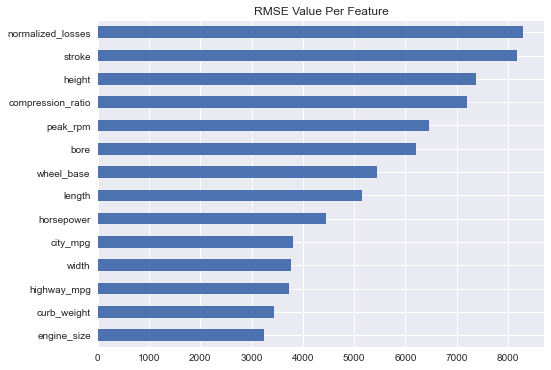After computing the RMSE value for each of the individual features, we can see that the top 5 features were the:

1. engine_size
2. curb_weight
3. highway_mpg
4. width
5. city_mpg

## Hyperparameter Optimisation¶

We are going to vary the k value of our model between 1 and 9 and see how it performs at each k value. We will compute the average RMSE values of each of the features for each of the k values.

In :
def knn_train_test(cols, col2, df, k=5):
''' Predict a variable and calculate RMSE value.

This function takes in a list of columns to train, the target column to predict, and a DataFrame,
randomizes the index and then uses the scikit-learn.neighbors KNeighborRegressor class
to predict a variable and also uses scikit-learn.metrics.mean_square_error
to calculate the RMSE value by taking the squareroot of the mean square error.

Parameters
----------
cols : list
list of columns in DataFrame to train.
col2 : str
name of target column in DataFrame.
df : DataFrame
n : int, default 5
number of n_neighbors.

Returns
-------
predictions : numpy.ndarray
numpy array with the predicted values of target column.
rmse : float
squareroot of mean_squared_error.
'''
np.random.seed(1) # random seed set to one so shuffling can be recreated
shuffle_index = np.random.permutation(df.index)
rand_df = df.reindex(shuffle_index)
split_index = int(df.shape / 2) # split the DataFrame index in 2
train_df = rand_df.iloc[:split_index]
test_df = rand_df.iloc[split_index:]

model = KNeighborsRegressor(n_neighbors = k)
model.fit(train_df[cols], train_df[col2])
predictions = model.predict(test_df[cols])
mse = mean_squared_error(test_df[col2], predictions)
rmse = np.sqrt(mse)

return rmse, predictions

In :
n_neighbors = [1, 3, 5, 7, 9]
rmse_values = dict()

for col in all_features:
values_dict = {}
for k in n_neighbors:
rmse, predictions = knn_train_test([col], 'price', num_cars, k)
values_dict[k] = rmse

rmse_values[col] = values_dict

rmse_values


Out:
{'normalized_losses': {1: 7326.341301768571,
3: 6986.552764895337,
5: 8291.523385820003,
7: 7708.952227449723,
9: 7942.7827438607965},
'wheel_base': {1: 4616.855696250425,
3: 5242.4142516168595,
5: 5443.857347028851,
7: 5509.9977571942545,
9: 5435.529878198641},
'length': {1: 6487.918747179253,
3: 6134.085873035833,
5: 5150.4491768776825,
7: 4982.967035383064,
9: 4931.887957197089},
'width': {1: 5713.616311059048,
3: 4175.369845095562,
5: 3773.135498600601,
7: 3486.0353809367534,
9: 3508.8770012640794},
'height': {1: 10910.35914761746,
3: 7805.349593138741,
5: 7380.628859304605,
7: 7272.536203121596,
9: 7121.205659190993},
'curb_weight': {1: 4390.877922466076,
3: 3668.3589437240184,
5: 3439.4916393560256,
7: 3174.0695108639184,
9: 3373.692477825783},
'engine_size': {1: 3398.1555291069303,
3: 3143.6417681833063,
5: 3247.180990459263,
7: 3058.254836187008,
9: 3141.6475726897906},
'bore': {1: 5926.514010782393,
3: 5927.911351770069,
5: 6206.9245622933095,
7: 6239.1231894606235,
9: 6407.868283419558},
'stroke': {1: 6674.97957524965,
3: 6907.209119141793,
5: 8184.186085763203,
7: 8641.925187026514,
9: 7880.150619925742},
'compression_ratio': {1: 7344.202947903877,
3: 5943.417906577177,
5: 7193.5885991346495,
7: 7540.580427735179,
9: 7180.410828835924},
'horsepower': {1: 4183.096630487993,
3: 4176.022383933198,
5: 4456.175620282486,
7: 4658.0497482468845,
9: 4591.4584980438685},
'peak_rpm': {1: 8792.216444674234,
3: 7080.746020410247,
5: 6458.378473332141,
7: 6544.40112284804,
9: 6686.435851634613},
'city_mpg': {1: 4170.034286429789,
3: 3438.0091973569693,
5: 3813.193148373158,
7: 3706.737982542668,
9: 3811.0390099593706},
'highway_mpg': {1: 3667.7066335790814,
3: 3602.7490403548477,
5: 3737.1620469013646,
7: 3963.0230166902043,
9: 3972.4730855050143}}
In :
for k,v in rmse_values.items():
x = list(v.keys())
y = list(v.values())
# displays a scatter plot of the rmse values of the different features for n number of neighbors
plt.scatter(x,y,)
plt.xlabel('n_neighbor value')
plt.ylabel('RMSE')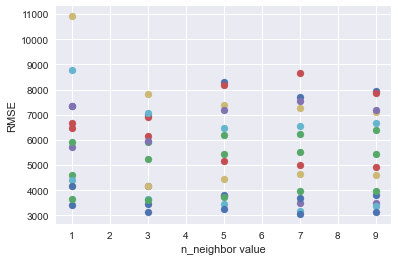There is no clear pattern to how the model behaves as we vary the values for k. For most features the RMSE values decreased as we varied k from 1 - 2, while in some features the RMSE increased as we varied k from 1 - 2.

In :
feature_avg_rmse = {}
for k,v in rmse_values.items():
avg_rmse = np.mean(list(v.values())) # computes the mean for the list of values in feature_avg_rmse
feature_avg_rmse[k] = avg_rmse
series_avg_rmse = pd.Series(feature_avg_rmse) # converts feature_avg_rmse dict to a pandas Series
sorted_series_avg_rmse = series_avg_rmse.sort_values() # sorts values in Series in ascending order
sorted_series_avg_rmse

Out:
engine_size          3197.776139
curb_weight          3609.298099
city_mpg             3787.802725
highway_mpg          3788.622765
width                4131.406807
horsepower           4412.960576
wheel_base           5249.730986
length               5537.461758
bore                 6141.668280
compression_ratio    7040.440142
peak_rpm             7112.435583
normalized_losses    7651.230485
stroke               7657.690117
height               8098.015892
dtype: float64
In :
sorted_series_avg_rmse.plot.barh(figsize=(8, 6)) # horizontal bar plot of sorted_Series_avg_rmse
plt.title('Average RMSE Value Per Feature')
plt.show()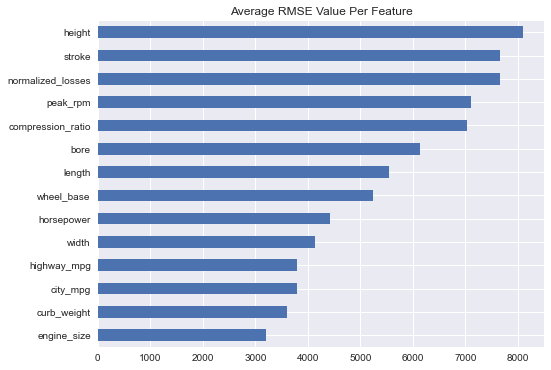After taking the average RMSES for each feature for the different k value, there is not so much change to the top 5 performing features. Just a slight difference in how it was ordered. The top 5 features are:

1. engine_size
2. curb_weight
3. city_mpg
4. highway_mpg
5. width

## Multivariate Model Testing¶

In our previous testing we used just one feature. Here we are going to use multiple features from the 5 best performing features in the last test. We are going to see how the model performs when we train it with the 2 best features up to the 5 best features.

In :
best_features = list(sorted_series_avg_rmse.index)
best_rmse = {}

for i in range(2, 6):
rmse, predictions = knn_train_test(best_features[:i], 'price', num_cars)
best_rmse[f'best {i} features'] = rmse

best_rmse

Out:
{'best 2 features': 2776.7887244801323,
'best 3 features': 3007.3486027396293,
'best 4 features': 3207.3124028694183,
'best 5 features': 2660.144132034954}

The model performed best when we used the best 2 features, best 3 features and best 5 features. In fact we got the lowest RMSE score when we used the best 5 features.

## Multivariate Hyperparameter Optimisation¶

We are going to vary the k value between 1 - 25 for the 2 best , 3 best and 5 best features.

In :
top3_features = [2, 3, 5]
top3_rmse = {}

for i in top3_features:
top_rmse = {}
for k in range(1, 26):
rmse, predictions = knn_train_test(best_features[:i], 'price',
num_cars, k)
top_rmse[k] = rmse
top3_rmse[f'best {i} features'] = top_rmse

top3_rmse


Out:
{'best 2 features': {1: 3405.0011057266925,
2: 2806.815926009399,
3: 2832.046659416315,
4: 2755.885691343166,
5: 2776.7887244801323,
6: 2845.331496943722,
7: 2939.963979267051,
8: 3090.0602294645896,
9: 3287.39623079902,
10: 3425.1888036865944,
11: 3584.4292620828824,
12: 3635.5259878023303,
13: 3663.6037412497835,
14: 3664.5333259279732,
15: 3681.5923927742647,
16: 3707.625229104499,
17: 3740.66261656526,
18: 3711.4044350521394,
19: 3747.9428945198347,
20: 3731.3753714046247,
21: 3721.4542332692286,
22: 3754.542140976416,
23: 3768.9635970788922,
24: 3805.6710862613386,
25: 3838.1666278221946},
'best 3 features': {1: 2777.6202422217475,
2: 2606.028364197136,
3: 2804.9334531618865,
4: 2973.8428185388348,
5: 3007.3486027396293,
6: 3164.6180440931576,
7: 3320.9176540989574,
8: 3227.3661070455346,
9: 3222.521540580632,
10: 3381.7607558637264,
11: 3514.6647126805688,
12: 3478.188779838502,
13: 3467.197769280226,
14: 3425.761838598229,
15: 3481.529833666676,
16: 3526.896765217264,
17: 3561.375357409941,
18: 3554.9756094603554,
19: 3567.7774424939935,
20: 3613.753863906616,
21: 3681.0256070089736,
22: 3750.5443211674387,
23: 3799.0855320622077,
24: 3840.076380126876,
25: 3927.030598603479},
'best 5 features': {1: 2520.631347500066,
2: 2485.4355156591773,
3: 2763.9154195934916,
4: 2686.6582848168464,
5: 2660.144132034954,
6: 2600.58531392454,
7: 2688.8790008523047,
8: 2639.4963255917783,
9: 2800.6577554805467,
10: 2959.364188064727,
11: 3122.290167118673,
12: 3282.190204567422,
13: 3428.774639756408,
14: 3489.746757536239,
15: 3609.77239905479,
16: 3682.175611900524,
17: 3709.2126092691487,
18: 3761.0485768435037,
19: 3830.139389636404,
20: 3848.204112949962,
21: 3917.2545706083974,
22: 3954.1214636473746,
23: 4011.3923030465867,
24: 4056.2210950690715,
25: 4083.7093746151913}}
In :
for k,v in top3_rmse.items(): # scatter plot for each of the rmse values and n_neighbor values of the top 3 performing multi features
x = list(v.keys())
y = list(v.values())

plt.scatter(x,y, label=f'{k}')
plt.xlabel('n_neighbor value')
plt.ylabel('RMSE')
plt.legend()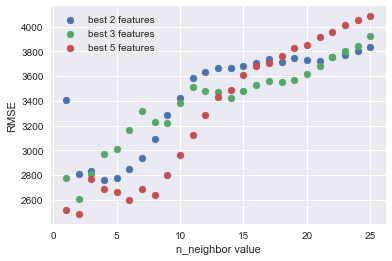The RMSE values dropped as the n_neighbor value varied from 1 - 2 and then alternated a little bit before climbing steadily as the value for the n_neighbor increased.

## Cross Validation¶

Cross validation is important as it prevents your model from overfitting. We have chosen to work with a maximum of 6 folds so that that sample from each fold is representative enough as there are not a lot of rows in our dataset.

In :
def crossval_train_test(cols, col2, df, n=2):
''' Calculates average rmse and std rmse value for n number of folds.

This function takes in a list of columns to train, the target column to predict, and a DataFrame
The DataFrame is split and randomized using scikit-learn.model_selection KFold class while the
average rmse and std rmse values are calculated by taking the mean and std of the squareroot of
mean_squared_error values returned by scikit-learn.model_selction cross_val_score class.

Parameters
----------
cols : list
list of columns in DataFrame to train.
col2 : str
name of target column in DataFrame.
df : DataFrame
n : int, default 2
number of splits.

Returns
-------
avg_rmse : float
average rmse value.
std_rmse : float
standard deviation of rmse values.
'''
kf = KFold(n, shuffle=True, random_state = 1)
model = KNeighborsRegressor()
mses = cross_val_score(model, df[cols],
df[col2], scoring = 'neg_mean_squared_error',
cv = kf)
rmses = np.sqrt(abs(mses))
avg_rmse = np.mean(rmses)
std_rmse = np.std(rmses)

return avg_rmse, std_rmse

In :
five_features = {}
for i in range(2,7):
avg_rmse, std_rmse = crossval_train_test(best_features[:5], 'price',
num_cars, i)
five_features[f'{i} folds'] = (avg_rmse, std_rmse)

five_features

Out:
{'2 folds': (3564.9159667286813, 900.4289986239398),
'3 folds': (3450.5535754697853, 844.1974363600241),
'4 folds': (3213.8019407419806, 740.0482772651251),
'5 folds': (3114.5331206578367, 1245.0075681397911),
'6 folds': (3025.0407445505807, 1293.1695693291022)}
In :
averages = []
stds = []
for k,v in five_features.items():
avg = v
std = v
averages.append(avg)
stds.append(std)

plt.figure(figsize=(8, 6))
x = np.arange(5)
width = 0.4
plt.xticks(x, ['2 folds', '3 folds', '4 folds', '5 folds', '6 folds'])
bar1 = plt.bar(x - width/2, averages,
label='avg rmse', width = width
)
bar2 = plt.bar(x + width/2, stds,
label='std rmse', width = width
)

plt.xlabel('number of folds')
plt.legend()
plt.title('Average RMSE and STD RMSE For Different Folds')
plt.show()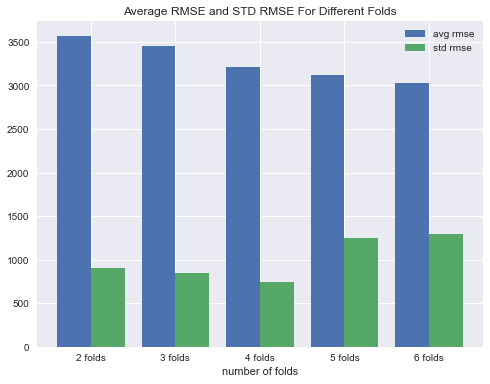The average RMSE values and the standard deviation of the RMSE values reduced as we varied the number of folds from 2 to 4. And when we varied from 4 folds up to 6 folds, the average RMSE values reduced still but the standard deviation of the RMSE values increased. Optimally we want a low bias(average RMSE value) as well as a low variance(standard deviation of RMSE) but there is usually a trade off between the bias and the variance.

## Conclusion¶

While our dataset was not large enough to make a concrete prediction, it was valuable process in learning a machine learning work flow. One of the things learned here is that we can improve the performance of the model by either increasing the number of features we train the model on or by varying the hyperparameters. It is also important to note that training the model on more features or increasing the number of hyperparameters(n_neighbours) does not neccesarily result in better performance of the model.